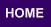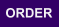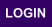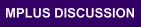Mplus
Thursday
August 18, 2022Mplus Mplus at a GlanceGeneral DescriptionMplus Programs Pricing Version History System Requirements Platforms
 Analyses/ResearchMplus Examples Papers References
 Search

MODELING WITH CATEGORICAL LATENT VARIABLES

Ellipse B describes models with only categorical latent variables. Following are models in Ellipse B that can be estimated using Mplus:

• Regression mixture modeling
• Path analysis mixture modeling
• Latent class analysis
• Latent class analysis with covariates and direct effects
• Confirmatory latent class analysis
• Latent class analysis with multiple categorical latent variables
• Loglinear modeling
• Non-parametric modeling of latent variable distributions
• Multiple group analysis
• Finite mixture modeling
• Complier Average Causal Effect (CACE) modeling
• Latent transition analysis and hidden Markov modeling including mixtures and covariates
• Latent class growth analysis
• Discrete-time survival mixture analysis
• Continuous-time survival mixture analysis

Observed outcome variables can be continuous, censored, binary, ordered categorical (ordinal), unordered categorical (nominal), counts, or combinations of these variable types. Most of the special features listed above are available for models with categorical latent variables. The following special features are also available:

Analysis with between-level categorical latent variables
• Test of equality of means across latent classes using posterior probability-based multiple imputations
• Plausible values for latent classes